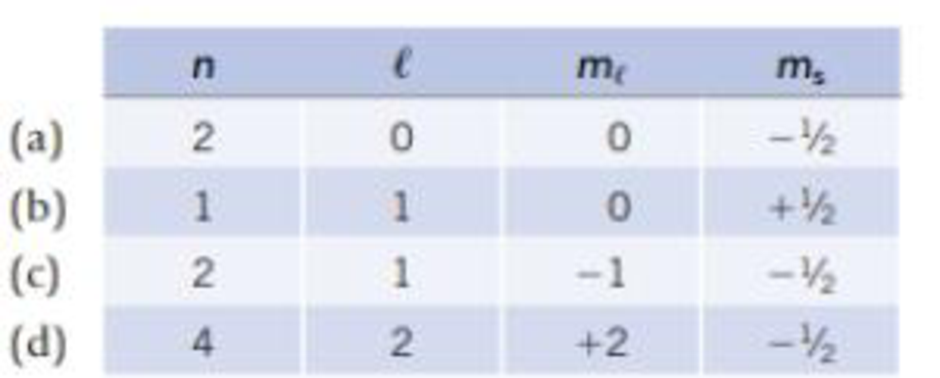# Which of the following is not an allowable set of quantum numbers? Explain your answer briefly. For those sets that are valid, identify an element in which an outermost valence electron could have that set of quantum numbers.### Chemistry & Chemical Reactivity

9th Edition
John C. Kotz + 3 others
Publisher: Cengage Learning
ISBN: 9781133949640

#### Solutions

Chapter
Section### Chemistry & Chemical Reactivity

9th Edition
John C. Kotz + 3 others
Publisher: Cengage Learning
ISBN: 9781133949640
Chapter 7, Problem 43GQ
Textbook Problem
76 views

## Which of the following is not an allowable set of quantum numbers? Explain your answer briefly. For those sets that are valid, identify an element in which an outermost valence electron could have that set of quantum numbers.Interpretation Introduction

Interpretation:

The allowable set of quantum numbers and an element in which an outermost valence electron could have that set of quantum numbers have to be identified and explained

Concept Introduction:

Electronic configuration: The electronic configuration is the distribution of electrons of an given molecule or respective atoms in atomic or molecular orbitals.

The important there rules for electronic configuration given below:

Aufbau principle: This rule statues that ground state of an atom or ions electrons fill atomic orbitals of the lowest available energy levels before occupying higher levels. If consider the 1s shell is filled the 2s subshell is occupied.

Quantum numbers: These terms are explained for the distribution of electron density in an atom. They are derived from the mathematical solution of Schrodinger’s equation for the hydrogen atom.  The types of quantum numbers are the principal quantum number (n), the angular momentum quantum number (l), the magnetic quantum number (ml) and the electron spin quantum number (ms). Each atomic orbital in an atom is categorized by a unique set of the quantum numbers.

### Explanation of Solution

Reason for the correct option:

• Option b:
• The principle quantum number: n = 1
• Azimuthal quantum number: l = 1, indicates the p-orbital.
• Magnetic quantum number: ml = 0, indicates orientation of p-orbitals in space of an orbital of an given energy (n) and shape (l). This number divides the subshell into individual orbitals which hold the electrons; there are 2l+1 orbitals in each subshell.

For,2l+1=21+1=3 The calculation results in the three sub-shell orbitals.

• Spin quantum number: ms = +1/2, indicates the orientation of intrinsic angular momentum of an electron in an atom as-1/2and+1/2. It describes the quantum state of an electron.

ms=+1/2Indicates the electron is located as up spin.

That is,

The magnetic number violates at the first principle shell no other orbital except s-orbital is present. Hence, the option is not valid. Hence, the in-valid option is b.

Therefore, the correct option is (b).

Reason for the in-correct options:

• Option a):
• The principle quantum number: n = 2
• Azimuthal quantum number: l = 0, indicates the s-orbital.
• Magnetic quantum number: ml = 0, indicates orientation of s-orbitals in space of an orbital of an given energy (n) and shape (l). This number divides the subshell into individual orbitals which hold the electrons; there are 2l+1 orbitals in each subshell.

For,2l+1=20+1=1 The calculation results in the one sub-shell orbital.

• Spin quantum number: ms = -1/2, indicates the orientation of intrinsic angular momentum of an electron in an atom as-1/2and+1/2. It describes the quantum state of an electron.

ms=1/2Indicates the electron is located as down spin.

That is,

2s1principlequantumnumber:2Azimuthalquantumnumber:smagneticquantumnumber:onesub-shellispresent.Spinquantumnumber:down-spin,

The orbital box diagram of the given information is; 2s1, the innermost orbital 1s2 is understood and the element that Lithium and Beryllium as the both contain outer most valence electron in 2s-orbital. Hence, the valid option is a.

• Option c:
• The principle quantum number: n = 2
• Azimuthal quantum number: l = 1, indicates the p-orbital.
• Magnetic quantum number: ml = -1, indicates orientation of s-orbitals in space of an orbital of given energy (n) and shape (l). This number divides the subshell into individual orbitals which hold the electrons; there are 2l+1 orbitals in each subshell.

For,2l+1=21+1=1 The calculation results in the one of the three sub-shell orbitals.

• Spin quantum number: ms = -1/2, indicates the orientation of intrinsic angular momentum of an electron in an atom as-1/2and+1/2. It describes the quantum state of an electron.

ms=1/2Indicates the electron is located as down spin.

That is,

2p1principlequantumnumber:2Azimuthalquantumnumber:pmagneticquantumnumber:oneofthethreesub-shellispresent

### Still sussing out bartleby?

Check out a sample textbook solution.

See a sample solution

#### The Solution to Your Study Problems

Bartleby provides explanations to thousands of textbook problems written by our experts, many with advanced degrees!

Get Started

Find more solutions based on key concepts
Bile is essential for fat digestion because it splits triglycerides into fatty acids and glycerol. emulsifies f...

Nutrition: Concepts and Controversies - Standalone book (MindTap Course List)

How can errors in the cell cycle lead to cancer in humans?

Human Heredity: Principles and Issues (MindTap Course List)

Assign IUPAC names to each of the following compounds.

General, Organic, and Biological Chemistry

What is meant by residence time? Does seawater itself have a residence time?

Oceanography: An Invitation To Marine Science, Loose-leaf Versin

An aluminum calorimeter with a mass of 100 g contains 250 g of water. The calorimeter and water are in thermal ...

Physics for Scientists and Engineers, Technology Update (No access codes included)

What are valence electrons?

Introductory Chemistry: An Active Learning Approach